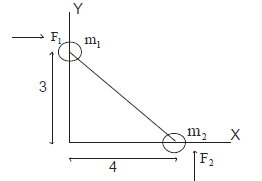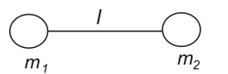# Center of mass problems with solutions

## Multiple Choice Questions

Question 1
A system consisting of two objects has a total momentum of (18 kgm/sec)i and its center of mass has the velocity of (3 m/s)i.One of the object has the mass 4 kg and velocity (1.5 m/s)i.The mass and velocity of the other objects are
(a) 2 kg, (6 m/s)i
(b) 2 kg, (-6 m/s)i
(c) 2 kg, (3 m/s)i
(d) 2 kg, (-3 m/s)i

Question 2
A moving bullet hits a solid target resting on a frictionless surface and get embedded in it.What is conserved in it?
(a) Momentum Alone
(b) KE alone
(c) Both Momentum and KE
(d) Neither KE nor momentum

Question 3
A stationary body of mass 3 kg explodes into three equal parts.Two of the pieces fly off at right angles to each other with the velocities 2i m/s and 3j m/s.If the explosion takes place in 10-3 sec.find out the average force action on the third piece in N
(a) (-2i-3j)103
(b) (2i+3j)103
(c) (2i-3j)10-3
(d) none of these

Question 4
Consider the following two statements.
STATEMENT A Linear Momentum of a system of particles is zero.
STATEMENT B Kinetic energy of system of particles is zero.
(a) A does not imply B and B does not imply A.
(b) A implies B but B does not imply A
(c) A does not imply B but B implies A
(d) A implies B and B implies A.

Question 5
The Position vector of the center of mass of uniform semi circular ring of radius R and Mass M whose center coincided with the origin
(a) r=(2R/π)j
(b) r=(R/π)j
(c) r=(4R/π)j
(d) none of the these

Question 6
Two electrons(e) are the at the point (-a,0) and (a,0).Their mass is m.They are released from rest.The acceleration of the center of mass of the system when the electron at 4a distance apart
(a) e2/64mπε0a2
(b) zero
(c) e2/16mπε0a2
(d) none of the above

Question 7
A system consisting of two objects has a total momentum of (18 kgm/sec)i and its center of mass has the velocity of (3 m/s)i.One of the object has the mass 4 kg and velocity (1.5 m/s)i.The mass and velocity of the other objects are
(a) 2 kg, (6 m/s)i
(b) 2 kg, (-6 m/s)i
(c) 2 kg, (3 m/s)i
(d) 2 kg, (-3 m/s)i

Question 8
An astronaut has just finished fixing a space telescope using a big instrument whose mass is one tenth as big as his mass. You realize you have no way to get back to your spaceship which is 10 meters away from you, so you throw the instrument as hard as you can in a direction away from the spaceship which causes you to move in the opposite direction, toward the spaceship. When you finally reach the space ship, how far away are you from the instrument? (a) 110m
(b) 100m
(c) 80m
(d) 90

Question 9
A grenade of mass M is falling with a velocity v0 at height when it explodes into two equal fragments that initially move horizontally in center of mass frame. The explosion is such that
$Q=KE_f - KE_i=mv_0^2$
Determine where the fragment will fall on the ground relative to the point directly below the grenade at the time of explosion
(a) $\frac {v_0 [\sqrt {v_0^2+2gh}-v_0]}{g}$
(b) $\frac {v_0 \sqrt 2[\sqrt {v_0^2+2gh}-v_0]}{g}$
(c) $\frac {2v_0 [\sqrt {v_0^2+2gh}-v_0]}{g}$
(d) None of the above

## Paragraph Based Questions

Two masses constrained to move in a horizontal plane collide. Given initially that M 1 = 85gm and  M2 = 200gm, v1 = 6.4i cm/sec and v2 = -6.7i - 2j cm/sec.
i and j are the unit vectors across x and y axis respectively
Question 10
Find the Velocity of Center of mass
(a) -2.8i - 1.4j
(b) 2.8i + 1.4j
(c) 2i + 1.4j
(d) none of the above

Question 11
Find the total Linear Momentum of the system
(a) -798i - 400j
(b) -796i + 400j
(c) 600i + 400j
(d) none of the above

Question 12
Find the velocity in the reference of frame in which centre of mass is at rest
(a) 9.2i - 1.4j ,- 3.9i - 0.6j
(b) 9.2i + 1.4j ,- 3.9i - 0.6j
(c) -9.2i + 1.4j, - 3.9i - 0.6j
(d) none of the above

Question 13
Let w1 and w2 are final velocity
now we know |w1| = 9.2 and w2 = 4.4i + 1.9j cm/sec
find the direction of w1
(a) 26° with respect to x axis
(b) 84° with respect to x axis
(c) -84° with respect to x axis
(d) none of the above

## Multiple Choice Questions

Question 14
A straight rod of length L has one of its end at origin  and other at (L, 0). If the mass per unit length of rod is  Ax + B .Find the centre of mass
(a) L(2AL + 3B)/(3AL + 6B)
(b) L(AL + 3B)/(AL + 6B)
(c) L(AL - B)/(AL + 2B)
(d) none of the above

Question 15
A uniform solid sphere has a spherical hole in it. Find the centre of mass
(a) xcm = -a3b/(R3-a3), ycm = 0, zcm = 0
(b) xcm = a3b/(R3-a3), ycm = 0, zcm = 0
(c) xcm = b3a/(R3-a3), ycm = 0, zcm = 0
(d) none of the above

## Paragraph Based Questions

(B) Two mass m1= 10 and m2 = 6 are joined by a rigid bar of negligible mass as shown in FigureBeing initially at rest, they are subjected to force F1 = 8i N and F2 = 6j N

Question 16
Find the coordinate of their centre of mass as a function of time
(a) i(1.5 - 0.25t2) + j(1.88 - 0.188t)
(b) i(1.5 + 0.25t2) + j(1.88 + 0.188t2)
(c) (1.5 + 0.188t2) i
(d) none of the above

Question 17
Find the total linear momentum as function of time
(a) (8i + 6j)t
(b) (8i -6j)t
(c) (2i +6j)t
(d) none of the above

(C) A mass of man m standing on a block of mass M. The system is at rest. The man moves relative in x direction to the block with velocity v' and then stops.

Question 18
Find the Velocity of Center of mass
(a) v'
(b) 0
(c) $\frac {mv'}{M+m}$
(d) None of the above

Question 19
Find the displacement of block relative to ground if the displacement of the man with respect to block is xi
(a) $\frac {-mx}{M+m}\mathbf{i}$
(b) $\frac {mx}{M+m}\mathbf{i}$
(c) xi
(d) none of the above

Question 20
Find the horizontal component of the force with when acted on the block M  during the motion
(a) $\frac {-mM}{M+m} \frac {dv'}{dt}\mathbf{i}$
(b) $-\frac {M+m}{Mm} \frac {dv'}{dt}\mathbf{i}$
(c) $m \frac {dv'}{dt} \mathbf{i}$
$(d) none of the above (D)A system is composed of two particles having mass m1 and m2 respectively. They are connected by a light spring of spring constant K. At time t=0, the system is thrown in the air such that m1 has initial velocity v1 and m2 has initial velocity v2 . Neglect any air drag in the motion Question 21 Find the total momentum of the system at time t (a)$m_1\mathbf{v_1}+m_2\mathbf{v_2}+(m_1+m_2)\mathbf{g}t$(b)$m_1\mathbf{v_1}+m_2\mathbf{v_2}+\mathbf{g}t$(c)$m_1\mathbf{v_1}+m_2\mathbf{v_2}$(d) None of these Question 22 Find the radius vector of the center of the mass relative to initial position. (a)$\frac{1}{2}\mathbf{g}t^2$(b)$\frac{m_1\mathbf{v_1}+m_2\mathbf{v_2}}{m_1+m_2}t+\frac{1}{2}\mathbf{g}t^2$(c)$(m_1\mathbf{v_1}+m_2\mathbf{v_2})t$(d) None of these ## Multiple Choice Questions Question 23 Two masses m1 and m2 separated by thin rod of length L. The system is shown belowThe rod mass can be neglected The system is thrown into the air. It is assumed only gravitational forces are acting and frictional forces are assumed to be null Which of the following statement is true? (a) The center of mass of the system will follow a parabolic path (b)$\frac{d\mathbf{p}_{total}}{dt}=m_1\mathbf{g+}m_2\mathbf{g}\$
(c) The acceleration of the center of mass will be vertically downward and equal to g
(d) None of these

## Subjective Questions

Question 24
10 objects of mass m0,2 m0,3 m0………………….10 m0 are placed on the x axis at
(L,0),(2L,0)……(10L,0).Find the Center of mass of the system